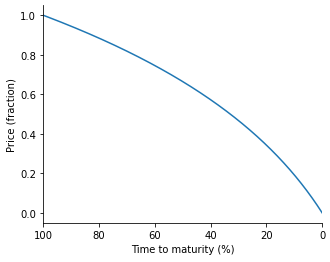# Pendle AMM

The Pendle AMM aims to minimize time-dependent impermanent loss that arises from the provision of liquidity using tokens with time decay.

# The Math Behind Pendle’s AMM

The Pendle AMM observes the formula

where

ɑ: the weight of x at time = i + 1 β: the weight of y at time = i + 1 x$_i$ and y$_i$: the equilibrium point of x and y at time = i

At time = 0, ɑ and β are initiated at 0.5, the quantity of Token X and Token Y is initiated by the market creator which translates to x$_0$ and y$_0$. The curve will resemble Uniswap’s constant product curve.

When a swap happens, the equilibrium point shifts along the curve.

At subsequent time step time = i, ɑ and β will change with the following formulas:

where

t: time to maturity from 1 to 0 T: UNIX timestamp T$_{start}$: contract start timestamp T$_{end}$: contract end timestamp

The changing weights of X and Y cause changes in the shape of the curve.

The pivot point for curve shifting is the equilibrium point of X and Y at the time of curve shift. Swapping between X and Y changes the equilibrium point, and the pivot point for curve shift will vary accordingly.

At time close to T$_{end}$, the slope of the curve will be near zero. And reaches zero at T$_{end}$.

# Curve Shifting

The Pendle curve shifting algorithm closely resembles the concept of option pricing models, where price decays more drastically towards the end of the contract than at the beginning. The magnitude of price decay over time is represented by the p(t) formula outlined in the section above.

The motivation for the constants chosen for the above formula is the fact that the price loses about 1/3 of its original value (at the beginning of the contract period) when time to maturity reaches 50%.The function of price (fraction) w.r.t. time remaining to maturity

# Fees

There will be a default 0.35% liquidity provider fee for all trades against liquidity pools on the Pendle's AMM. This fee will be adjustable via governance proposals and voting once it goes live.

The Pendle AMM is designed to support a protocol-level exit fee to be used to support continual work on the Pendle platform. However, it is currently being set to 0% and will be changeable via governance proposals if deemed necessary.

# LP Token Issuance/Burning

The LP token issuance will be in proportion to the liquidity provided to the pool.

## Liquidity Provision

Taking inspiration from Balancer, for a generic joinMarketByAll, the LP tokens received by the depositor is the ratio of deposited tokens to the current reserves of the token in the pool. In other words:

derives to

As mentioned, the swapFee variable will have to be introduced in the case of joinMarketSingleToken. In other words, the formula for LP token calculation for single token pool join is then (adapting from Balancer):

where

B$_{in}$: inTokenReserve.balance W$_{in}$: inToken Reserve.weight

## Liquidity Withdrawals

Without considering the swapFee, liquidity withdrawal formula is the inverse of that provision (without swapFee considerations).

With swapFee, adapting from Balancer, the formula for outAmountToken given inAmountLp is:

where

B$_{out}$: outTokenReserve.balance W$_{out}$: outTokenReserve.weight